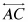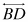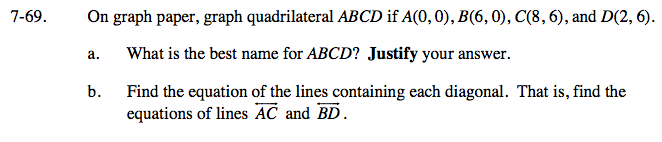### Home > GC > Chapter 7 > Lesson 7.2.3 > Problem7-69

7-69.
1. On graph paper, graph quadrilateral ABCD if A(0, 0), B(6, 0), C(8, 6), and D(2, 6). 7-69 HW eTool (Desmos). Homework Help ✎

2. Find the equation of the lines containing each diagonal. That is, find the equations of linesand.